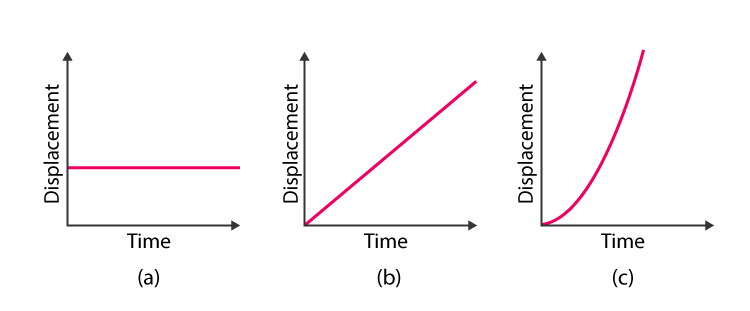# CBSE Class 9 Science Chapter 8 Revision Notes

Chapter 8: Motion Revision Notes

• When an object moves over time, it is said to be in motion.

• When a body moves uniformly, it covers the same distance in the same amount of time.

• When a body travels an unequal distance in the same amount of time. It moves in a non-uniform manner.

• In explaining motion, both distance and time are relevant.

• Even if you don’t see anything, you can tell when a motion has occurred.

• When two objects move in a plane (either in the same direction or in the opposite direction), each has a relative motion to the other.

For example, if a person is sitting on a train and watching a tree, the tree is steady but is assumed to be moving in relation to the train.

Displacement vs. Distance

• The distance travelled by an object.
• It only has magnitude and no direction.
• Displacement refers to how far and how far an object has moved from its initial position. i.e. the straight distance between the starting and ending places.

Speed

• The distance travelled by an object in a certain amount of time is called speed.
• Distance divided by time equals speed.
• The metric unit of speed is the metre per second (m/s).

Different Speeds

• Constant speed means that the speed does not alter.
• Changing speeds: 5 kilometres on a bicycle. Take off and pick up speed, slow down up hill, speed up down hill, and come to a complete stop at the stop sign. It took you 15 minutes to complete the journey (.25 h)
• Total speed divided by total time equals average speed.
• Speed at any given time is referred to as instantaneous speed.

Velocity

• Velocity is a term that encompasses both speed and direction.
• The storm is moving at a speed of 20 kilometers per hour.
• Assume that two trains are travelling at the same speed but in opposite directions, resulting in different velocities.
• A race car travelling around an oval track may maintain a constant speed yet have varying velocities at different points.

Acceleration

• Acceleration is defined as a change in velocity over a period of time.
• The direction is indicated by the (+ or -) sign. The + symbol indicates acceleration, while the – sign indicates deceleration.

Equation of uniform (constant) acceleration

a = v/t a = v/t a = v/t

• Galileo Galilei was an Italian physicist and astronomer who lived from 1564 to 1642. Formulated laws of motion for free-falling objects.
• Any object traveling freely under the effect of gravity alone is referred to as a freely falling object.
• It is independent of the object’s initial motion.
• Regardless of the beginning velocity, the acceleration of an item in free fall is downward.
• The acceleration of free fall (gravitational acceleration) is g = 9.80 m/s
• With rising altitude, g lowers.
• g is affected by latitude, height, and depth below the earth’s surface.
• 9.80 metres per second square is the average on the surface of the Earth
• The acceleration owing to gravity will be represented by the italics g.
• Not to be confused with the letter g, which stands for grams.
• Falling items can be regarded freely falling if there is no air resistance. Objects of various forms accelerate at varying rates (stone vs feather)
• Both upward and downward acceleration is possible.
• The journey follows a symmetrical pattern.
• The rate of acceleration is constant.
• At identical heights, the magnitude of the velocities is the same.
• As time passes, the images get closer together.
• When the ball moves upward, the acceleration and velocity are in opposite directions.
• The rate of acceleration is consistent (violet arrows maintain the same length)
• In an upward motion, the velocity is decreasing (red arrows are getting shorter)
• Negative acceleration and positive velocity
• At maximum height, velocity is zero.
• The time it takes to get upward and back is always the same.

## GRAPHICAL REPRESENTATION OF MOTION

Graph of Distance and TimeSource:

• Distance-Time graphs depict how an object’s position changes over time.
• Non-linear fluctuations imply non-uniform motion, while linear variations imply uniform motion.
• We gain speed because of the slope.

Graph of Distance vs. Time

• Because the slope is constant, OA implies uniform motion with constant speed.
• Because the slope is zero, AB denotes that the body is at rest.
• The motion from B to C is non-uniform.

Graph of Velocity and Time

• The change in velocity with respect to time is represented by a velocity-time graph.
• Acceleration is provided by the slope.
• The displacement is determined by the area under the curve.
• Constant velocity is implied by a line parallel to the x-axis.

## EQUATIONS OF MOTION

Three equations can be used to describe the motion of an item travelling with uniform acceleration, namely

v= u + at

v2 – u2 = 2as

s = (1/2)at2 + ut

## UNIFORM CIRCULAR MOTION

• Uniform circular motion occurs when an object moves in a circular route at a constant speed.
• As the direction shifts, so does the velocity.
• The rate of acceleration is constant.

Source:

]]>